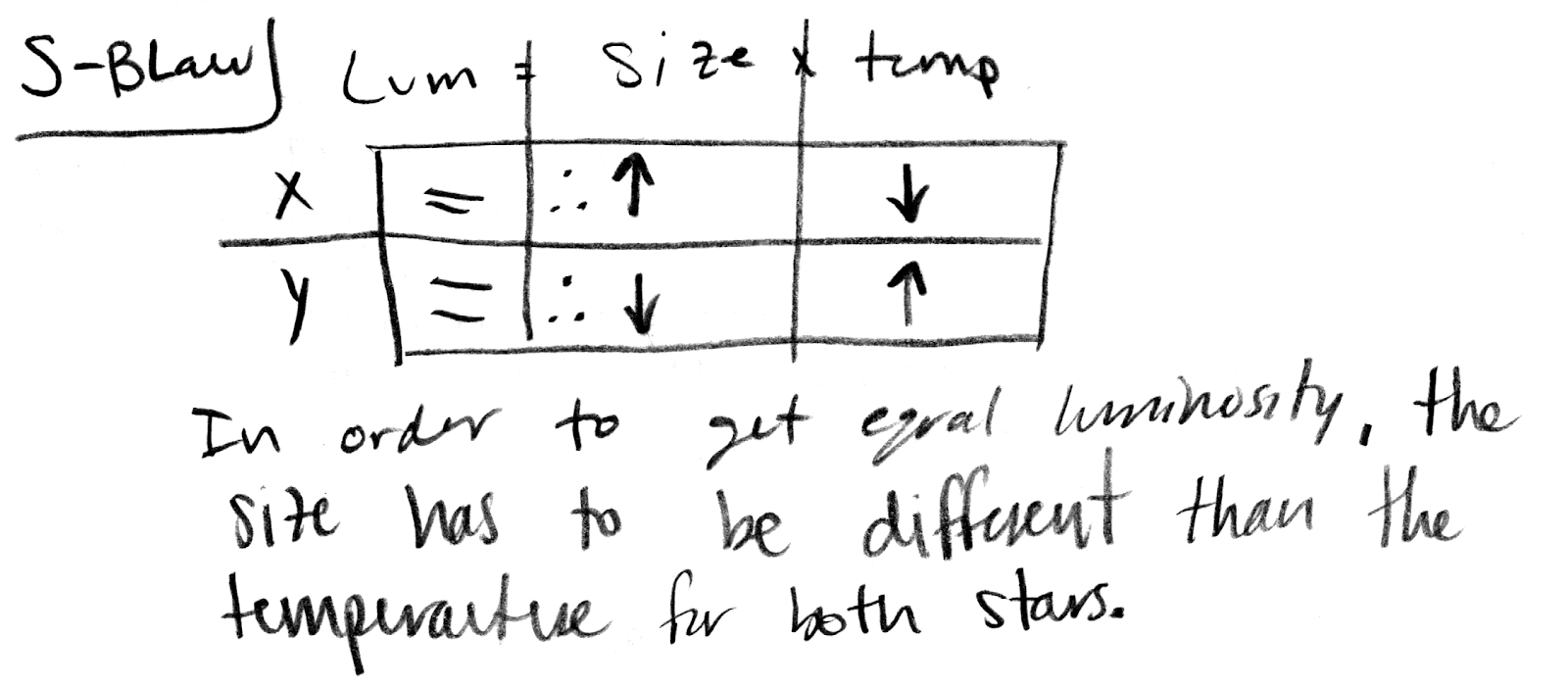## 20191122

### Astronomy midterm question: comparing sizes, temperatures of same-luminosity stars

Astronomy 210 Midterm 2, fall semester 2019
Cuesta College, San Luis Obispo, CA

The following claim was made by a student on an astronomy exam[*]:
2881: If two stars have the same luminosity, the star with the lower temperature must be larger.
Discuss whether this claim is correct or incorrect, and how you know this. Explain using Wien's law, the Stefan-Boltzmann law and/or an H-R diagram.

[*] waiferx.blogspot.com/2009/05/astronomy-midterm-question-cooler.html.

• p:
Correct. Discusses how the H-R diagram and/or the Stefan-Boltzmann law (luminosity is proportional to size × Temperature4) demonstrates that in order for a cooler star to have the same luminosity as a hotter star, its lower temperature must be compensated for by having a larger size; thus the claim by that student is correct.
• r:
Nearly correct (explanation weak, unclear or only nearly complete); includes extraneous/tangential information; or has minor errors.
• t:
Contains right ideas, but discussion is unclear/incomplete or contains major errors.
• v:
Limited relevant discussion of supporting evidence of at least some merit, but in an inconsistent or unclear manner. At least attempts to use Wien's law, the Stefan-Boltzmann law, and/or H-R diagram. May have argument based on the size of a star being dependent on luminosity and temperature.
• x:
Implementation/application of ideas, but credit given for effort rather than merit. Discussion not clearly based on Wien's law, the Stefan-Boltzmann law, and/or H-R diagram.
• y:
Irrelevant discussion/effectively blank.
• z:
Blank.
Section 70158
Exam code: midterm02Rf0w
p: 31 students
r: 1 student
t: 0 students
v: 1 student
x: 1 student
y: 0 students
z: 0 students

Section 70160
Exam code: midterm02T4qz
p: 16 students
r: 2 students
t: 0 students
v: 2 students
x: 2 students
y: 0 students
z: 0 students

A sample "p" response (from student 0809), using the Stefan-Boltzmann law:A sample "p" response (from student 1234), using a Hertzsprung-Russell diagram:A sample "p" response (from student 1278) using both the Stefan Boltzmann law and a Hertzsprung-Russell diagram:A sample "x" response (from student 4000), appealing to concepts other than than of the Stefan-Boltzmann law: